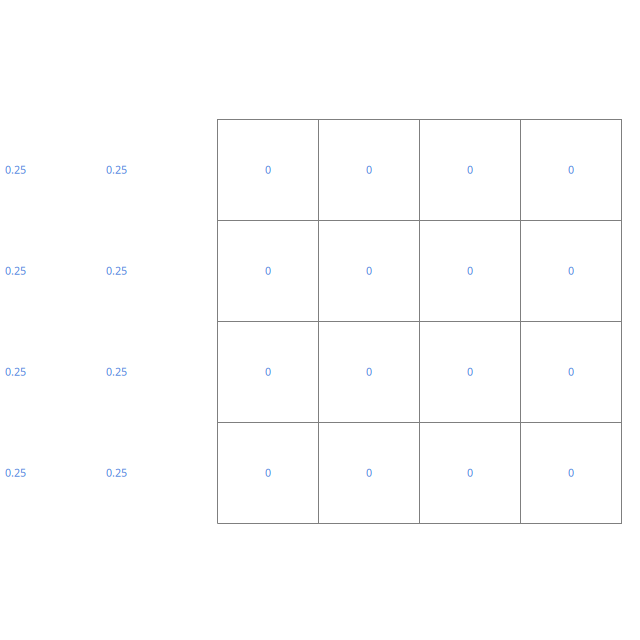# MWE: second row of ghost cells is wrong with neumann conditions

#define BGHOSTS 2

This minimum working example show that the command lines s[left] = neumann(a) followed by boundary ({s}) imposes the same value in the first and second row of ghost cells.

int main()
{
init_grid (4);
output_cells (stdout);

scalar s[];

s[left] = neumann(1.);

foreach()
s[] = 0.;

boundary ({s});

foreach()
fprintf (stderr, "%g %g %g\n", x, y, s[]);
foreach_boundary(left)
for (int i = -2; i < 0; i++)
fprintf (stderr, "%g %g %g\n", x + i*Delta, y, s[i]);
}

# Outputs

In the second row of cells, the value of s should be 0.5 instead of 0.25. This comes from the definition of the neuman command in common.h:

@define neumann(x) (Delta*(x) + val(_s,0,0,0))

which does not distinguish the first and second row of ghost cells and I do not see how to do it. I didn’t find where boundary() is defined and I don’t know how to test if we are in the first or second row of ghost cells.


blue="#5082DC"
set terminal @PNG enhanced size 640,640 font ",8"
set output 'boundary.png'
unset key
unset border
unset tics
unset xlabel
unset ylabel
set size ratio -1

plot 'out' w l lc rgb "#7F7F7F", \
'log' u 1:2:3 with labels tc rgb blueSecond row of ghost cells is wrong (script)